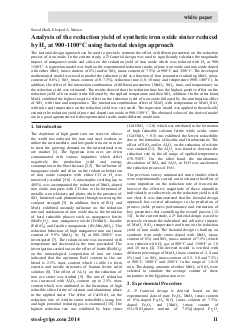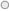# Analysis of the reduction yield of synthetic iron oxide sinter reduced by H² at 900-1100°C using factorial design approachThe factorial design approach can be used to precisely estimate the effect of different parameters on the reduction process of iron oxide. In the current study, a 24 factorial design was used to significantly calculate the magnitude impact of manganese oxide and silica on the reduction yield of iron oxide which was reduced with H2 at 900-1100°C. A regression model was built on the experimental reduction results of pure iron oxide and iron oxide doped with either MnO2 (mass content of 6%) and/or SiO2 (mass content of 7.5%) at 900°C and 1100°C. The developed mathematical model was used to predict the reduction yield as a function of four parameters including MnO2 (mass content of 0-6%), SiO2 (mass content of 0-7.5%), reduction time (1.0-10 min) and temperature (900-1100°C). In addition, the effect of the interaction combination of different parameters (MnO2, SiO2, time, and temperature) on the reduction yield was estimated. The results showed that the reduction time has the highest positive effect on the reduction yield of iron oxide sinter followed by the applied temperature and then SiO2 addition. On the other hand, MnO2 exhibited the highest negative effect on the reduction yield of iron oxide followed by the combination effect of SiO2 with time and temperature. The interaction combination effect of MnO2 with temperature or MnO2-SiO2 with time and temperature on the reduction yield was very small. The regression model was applied to theoretically estimate the reduction yield of pure and doped iron oxide at 900-1100°C. The obtained values of the derived model are in a good agreement with the experimental results under different conditions.